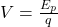## Which equation is used to calculate the electric potential in an electric field from a point charge? V = kq over d squared V = kq over d V

Question

Which equation is used to calculate the electric potential in an electric field from a point charge? V = kq over d squared V = kq over d V =delta U over q V = delta U over E

in progress 0
3 months 2021-07-25T17:36:49+00:00 2 Answers 6 views 0

The answer is V =delta U over q

Explanation:

Electric potential is defined as the magnitude of the electric field through the potential energy that a charge would have if placed at that point. Mathematically, the potential is defined with the following expression:where:

V is the electric potential. Its unit is Julius by Coulomb (J/C).

Ep is the electric potential energy that has a charge

q is the charge

In the question Ep = ΔU

Explanation:

The electrostatic potential is defined in terms of work done in taking a unit positive charge from infinity to the point in question.

V=kq/d

Where d= distance from infinity to the point in question

k= constant of proportionality

q= attracting charge

The electric potential is measured in volts